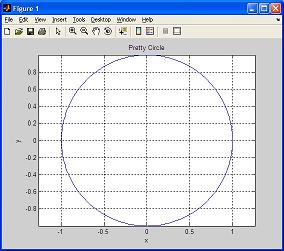# Tutorial Lesson: a Matlab Plot (creating and printing figures)

 You'll learn to make simple MATLAB plots and print them out. This lesson teaches you the most basic graphic commands. If you end an instruction with ';', you will not see the output for that instruction. MATLAB keeps the results in memory until you let the results out. Follow this example, step-by-step.

The video shows you the main idea. Below the video, you can see all the details of the code...

In the command window, you first create a variable named 'angle' and assign 360 values, from 0 to 2*pi (the constant 'pi' is already defined in MATLAB):

>> angle = linspace(0,2*pi,360);

Now, create appropriate horizontal and vertical values for those angles:

>> x=cos(angle);
>> y=sin(angle);

Then, draw those (x,y) created coordinates:

>> plot(x,y)

Set the scales of the two axes to be the same:

>> axis('equal')

Put a title on the figure:

>> title('Pretty Circle')

Label the x-axis and the y-axis with something explanatory:

>> ylabel('y')
>> xlabel('x')

Gridding the plot is always optional but valuable:

>> grid on

You now see the figure:The 'print' command sends the current plot to the printer connected to your
computer:

>> print

The arguments of the axis, title, xlabel, and ylabel commands are text strings. Text strings are entered within single quotes (').

Do you like your plot? Interesting and funny, isn't it?

From 'Matlab Plot' to home

From 'Matlab Plot' to 'Matlab Tutorial'